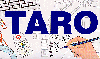The Traffic Accident Reconstruction Origin -Article-[Home] [ARnews] [Contents] [Classified] [Advertisers] [Approach Angles] [E-mail Directory] [Feedback] [Organizations] [Reference Library]

Appendix A

A Brief Discussion of Weight and Mass

The words mass and weight are often confused with each other. Weight and mass are two different concepts. The property a body has of resisting any change in its state of rest or uniform motion is called inertia. The inertia of a body is related to what we can think of as the amount of matter it contains. A quantitative measure of inertia is mass.* The SI unit of mass is the kilogram, and in the British system it is the slug. Unlike weight, mass is gravity independent. This means that if your mass is 5 slugs on Earth, it will be 5 slugs on any other planet, no matter what the gravity is.

Weight is the amount of attraction a body has to the earth because of its mass and the pull of gravity. A person who weighs 161 pounds is exerting a force of 161 pounds on the ground (and the ground is pushing back on your feet with an equal amount of force).

We can see how mass and weight is related by examining Newton’s second law, commonly written as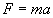, where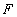is force,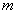is mass, and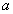is acceleration. Because weight is a force, we can substitute the letter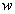(meaning weight) for. Now we have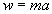. The acceleration in this formula is due to the pull of the earth on the body in question. We know that gravity is approximately 32.2 ft/sec/sec, so we substitute this number forand find that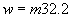. This equation tells us that we can easily convert from units of mass to weight, and vice versa, as long as gravity is about 32.2 ft/sec/sec. To solve for mass we rearrange the equation to read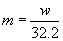.

In the case studies used in this paper, vehicle one’s mass was reported as 90 slugs. We can convert this to weight by multiplying by 32.2, as per the equation above. Therefore,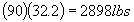.

* Beiser, Arthur. Applied Physics. Third ed. New York: McGraw Hill, 1994

[Home] [ARnews] [Contents] [Classified] [Advertisers] [Approach Angles] [E-mail Directory] [Feedback] [Organizations] [Reference Library]